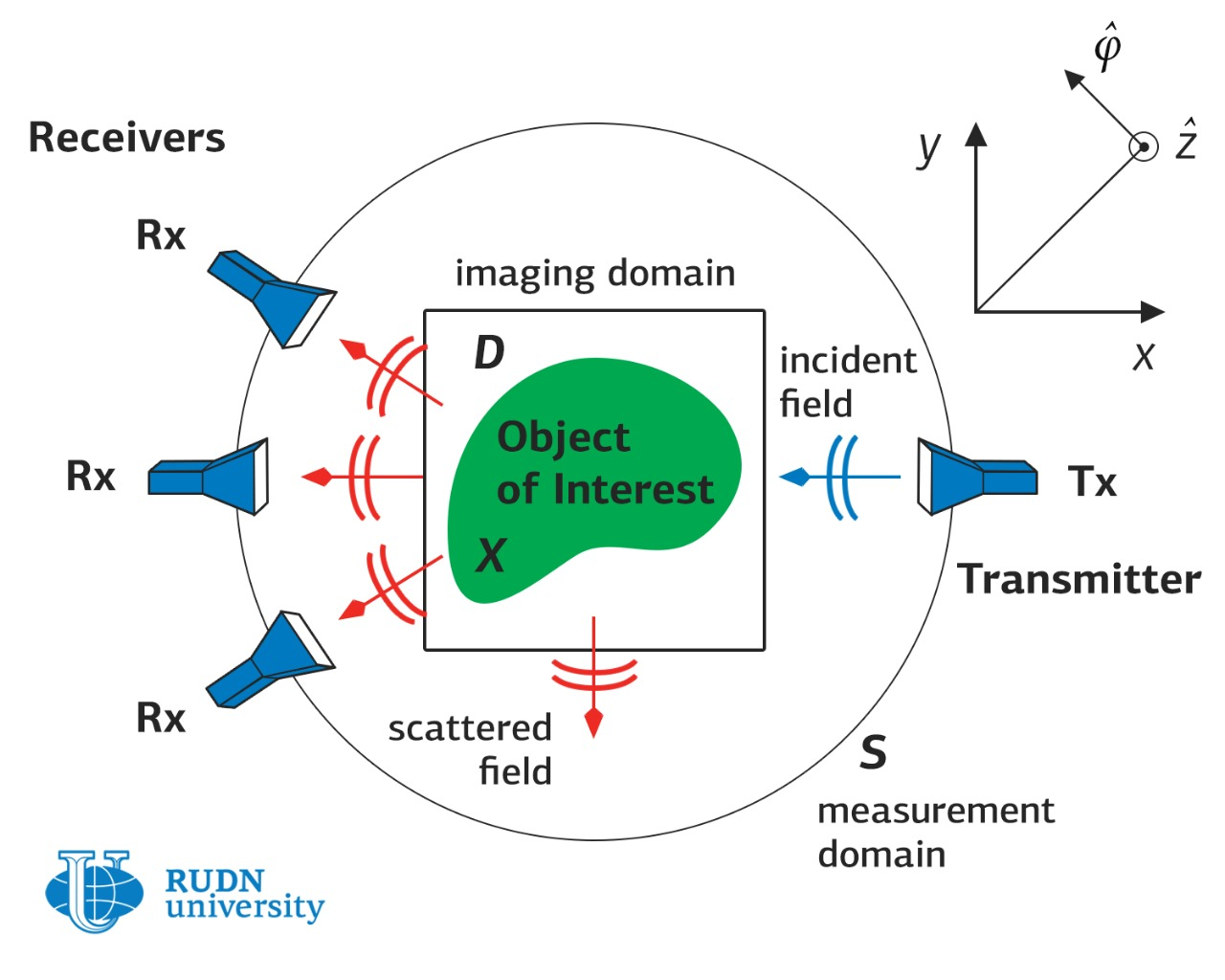RUDN University mathematician has developed a method of exploring the structure of molecules

# RUDN University mathematician has developed a method of exploring the structure of molecules

A scientist from the Mathematical laboratory of RUDN University has obtained new results in the study of the inverse problem for coupled Schrödinger equations. This result will be useful for describing the interaction of laser beams and particles with molecules and the analysis of molecular structures.

Usually, a mathematical problem is an equation that needs to be solved. But in physics, the opposite often happens: scientists know the solution — the results of measurements, but the properties of the physical system, that is, the equations that describe it, are unknown. This is called an inverse problem — the problem of finding an equation using its solution.

In quantum physics, it is often necessary to solve various variants of the inverse scattering problem, for example, to reconstruct the structure of a molecule using the scattering pattern of the particles with which it is fired. In this case, it is necessary to solve the Schrödinger equation for several particles, but this problem is not solved in general.

Therefore, it is necessary to find the measurements to reconstruct the potential uniquely, and create an algorithm by which the potential can be reconstructed numerically. Moreover, even if the numerical method has already been invented, you need to understand whether it is correct and whether it works as it should. Theorems evaluating the potential by measurements are needed to solve these problems.

QUOTE question 1: “Tell us about possible applications of your results for numerical methods”

Masahiro Yamamoto from RUDN University together with Fangfang Dou from China obtained such theorems. The case of coupled Schrödinger equations, previously not studied, was investigated in their research. In previous papers, inverse problems for ordinary and nonlinear Schrödinger equations have been studied. However, coupled Schrödinger equations are a relatively young class of problems. Therefore, their direct problem is investigated, while the reverse is not.

QUOTE question 2 “How do the practical applications of coupled nonlinear Schrödinger equations differ from the applications of your results? Can we expect your result to be applied to coupled nonlinear equations?”

Coupled Schrödinger equations are a system of two Schrödinger equations in which there are additional components responsible for the interaction of radiation and molecules. They are needed to describe recent experiments with the effect of laser radiation on intermolecular bonds in deuterium and hydrogen ions. Masahiro Yamamoto and Fangfang Dou obtained new theorems that allow estimating undisturbed radiation potentials using the results of measurements.

Their new study will allow applying numerical methods to models of multiphoton transitions, which will help us to simulate the change in the properties of chemical bonds under the influence of intense laser fields. Applications of these results for various studies in nanophotonics and mesoscopic physics are likely to arise in the future, because the question of controlling and suppressing the dissociation of molecules with laser radiation has been worrying physicists for a long time.

QUOTE question 3: “Tell us about the prospects for practical applications of numerical simulations for coupled Schrödinger equations”.

The article is published in Inverse Problems.News
All news
Science
13 Apr
RUDN University mathematician suggested new approach to cooperative game

A mathematician from RUDN University developed a matrix representation of set functions. This approach is vivid and easy to check, and it makes the calculations easier. Among other things, the new development can be applied to cooperative game theory.